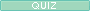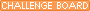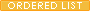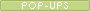myalgebra Ms. Standring Attleboro High School Send e-mailWelcome to our New Class Page!! :)     In our previous chapter, we were introduced to equations.  We learned how to solve and graph these equations.  Each equation we graphed was a line.  We will now look more closely at the graphs of such lines.
My Quia activities and quizzesSection 3:1 Question Sheet: session 3:1 questions
https://www.quia.com/quiz/521107.html
"Applying Rates" QuestionsSection 3:2 Question Sheet: session 3:2 questions
https://www.quia.com/quiz/521108.html
"Exploring Direct Variation" QuestionsSection 3:3 Question Sheet: session 3:3 questions
https://www.quia.com/quiz/521109.html
"Finding Slope" QuestionsSection 3:4 Question Sheet: session 3:4 questions
https://www.quia.com/quiz/521110.html
"Finding Equations of a Line" QuestionsSection 3:5 Question Sheet: session 3:5 questions
https://www.quia.com/quiz/521111.html
"Writing an Equation of a Line" QuestionsSection 3:6 Question Sheet: session 3:6 questions
https://www.quia.com/quiz/521112.html
"Modeling Linear Data" Questions"Graphing Linear Equations" Vocabulary
https://www.quia.com/jg/136612.html
Chapter Thee Vocabulary Game!Chapter Three Challenge
https://www.quia.com/cb/13866.html
Check your knowledge of Chapter Three!Section 3:5 Steps to Write an Equation
https://www.quia.com/rd/4222.html
Notes for Section 3:53:1 "Applying Rates"
https://www.quia.com/pop/13808.html
Get familiar with "Applying Rates"!3:2 "Exploring Direct Variation"
https://www.quia.com/pop/13810.html
Get familiar with "Exploring Direct Variation"!3:3 "Finding Slope"
https://www.quia.com/pop/13811.html
Get familiar with "Finding Slope"!3:4 "Finding Equations of a Line"
https://www.quia.com/pop/13812.html
Get familiar with "Finding Equations of a Line"!